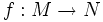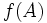# Nullset-preserving map

Let$M$ and$N$ be differential manifolds. A map$f:M \to N$ (not necessarily smooth) is termed nullset-preserving if$f(A)$ has measure zero if and only if$A$ has measure zero.